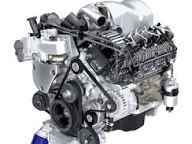Diesel EngineThe diesel engine was developed by Rudolf Diesel in 1892. This engine consists of a cylinder fitted with three-piston.Diesel engine PV-diagram

A heat engine with 100% efficiency is only a theoretical possible:
If Q1 is the amount of heat absorbed from the source and Q2 be the heat rejected to the sink, then the efficiency of the heat is given as

ɧ =

Since Q1> Q2, the efficiency, ɧ is always less than unity. Hence the efficiency of the heat engine cannot be 100%. Hence a heat engine with 100% efficiency is only a theoretical possibility.

Merits and demerits of diesel engine:

The diesel engine is very suitable for use in cars and jeep because its size is higher efficiency than a petrol engine. Its efficiency is quite high as compared to that of the petrol engine. This engine is more advantageous. Due to the high cost of petrol, the running of this engine is expensive so diesel is a better alternative.

The efficiency of the heat engine;

If Q1 is the amount of heat absorbed from the source and Q2 be the heat rejected to the sink, then the efficiency of the heat is given as

ɧ =

Since Q1> Q2, the efficiency, ɧ is always less than unity.

Heat cannot be completely converted into mechanical work:

If Q1 is the amount of heat absorbed from the source and Q2 be the heat rejected to the sink, then the efficiency of the heat is given as

ɧ =

Since Q1> Q2, the efficiency, ɧ is always less than unity. No engine will convert all the heat absorbed from the source into work. So heat cannot be completely converted into mechanical work.